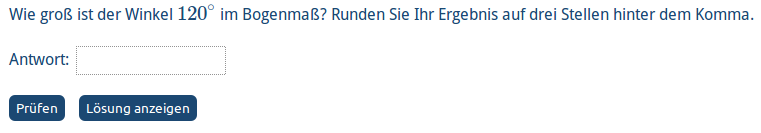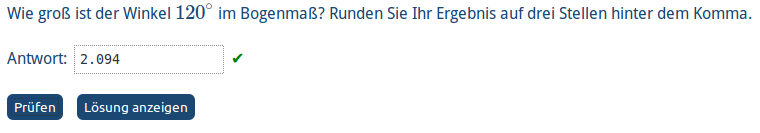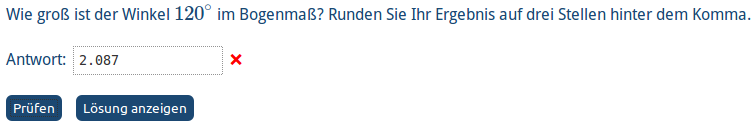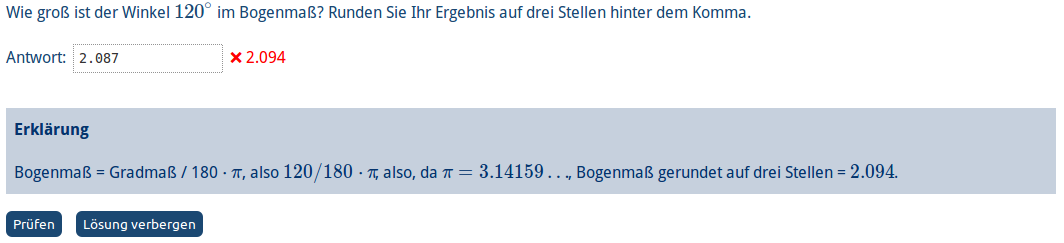Index

Working with MUMIE as author

• Initial steps:
• Articles:
• Problems:
• Programming with Python
• New Visualizations with JSXGraph
• Old Visualizations:
• Media Documents:

Working with MUMIE as teacher

Using MUMIE via plugin in local LMS

FAQ for examination lecturers

Overview

Go back to the old wiki

We revise and update this wiki. We apologize for the inconvenience this may cause you.

# Overview

Quick-Checks are small problems embedded in other documents. The user types the answer into one or more input fields,
and clicks a "Check" button to see whether the answer is correct or not.
The user can also click a "Show solution" button to see the solution and, if existing, an explanation.
In contrast to normal problems, no data is stored on the server, neither personalized data, nor answers, nor corrections.

Notice: For more than one language use only ONE Quick-Check. For each text use a seperate lang-environment (see below).

The reader of this document is supposed to have a basic knowledge of generic problems.

# TeX description

The TeX description of Quick-Checks is very similar to that of generic TeX problems. Here is an example:

123456789101112131415161718192021222324252627282930\begin{quickcheck}  \type{input.number}  \displayprecision{3}  \correctorprecision{3}   \begin{variables}    \randint{num}{1}{6}    \function[calculate]{deg}{num/6*180}    \function[calculate]{rad}{deg/180*pi}  \end{variables}   \lang{de}{\text{    Wie groß ist der Winkel $\var{deg}^\circ$ im Bogenmaß?    Runden Sie Ihr Ergebnis auf drei Stellen hinter dem Komma.     Antwort: \ansref}    \explanation{    Bogenmaß = Gradmaß / 180 $\cdot$ $\pi$, also $\var{deg}/180\cdot\pi$, also, da $\pi = 3.14159\ldots$,    Bogenmaß gerundet auf drei Stellen = $\var{rad}$.    }  \lang{en}{\text{...}  Answer: \ansref}  \explanation{...}  }    \begin{answer}    \solution{rad}  \end{answer}\end{quickcheck} 

In the browser, this looks as follows:If you type in the answer and click the check button, the system will mark correct and wrong answers as follows:Clicking the "Show solution" button will show the correct answer and, if existing, the explanation:The "Show solution" button changes to a "Hide solution" button by this, which hides these things again.

more examples

# Pools

Quick-Checks provide a functionality similar to random question pools in generic problems.
You can define a "pool" of Quick-Checks from which one or more are selected randomly. All Quick-Checks of the
pool must be enclosed in a quickcheckcontainer environment. The selection is controlled by one or
more \randomquickcheckpool commands, which are completely analog to the \randomquestionpool (see Randomquestionpool) commands in generic problems:

123456789101112131415\begin{quickcheckcontainer} \randomquickcheckpool{1}{5}\randomquickcheckpool{6}{10} \begin{quickcheck}  ...\end{quickcheck} \begin{quickcheck}  \explanation{...}\end{quickcheck}  ...\end{quickcheckcontainer} 

# List of TeX commands and environments

There are five Quick-Checks environments:

• quickcheck -- Top-level environment of a Quick-Checks
• quickcheckcontainer -- Container for Quick-Checks that should form a pool
• variables -- Container for variables; same as in generic problems
• choices -- multiple choice questions, usage: \begin{choice}{<type>}... where type either multiple or unique. The type yesno is not supported for quick checks yet. mc example
• choice -- one choice in a multiple choice questions

The Quick-Checks commands are:

• \ansref
• \checkAsFunction[<options>]{<variable>}{<min>}{<max>}{<steps>}
• \correctorprecision{<number>}
• \derivative[<actions>]{<variable>}{<expression>}{<free_variable>}
• \displayprecision{<number>}
• \explanation{...}
• \field{<field>}
• \function[<actions>]{<variable>}{<expression>}
• \number{<variable>}{<value>}
• \drawFromSet[<options>]{variable}{set}
• \precision{<number>}
• \randadjustIf{<variables>}{<condition>}
• \randdouble{<name>}{<min>}{<max>}
• \randint[<nonzero>]{<name>}{<min>}{<max>}
• \randomquickcheckpool{<min>}{<max>}
• \randrat{<name>}{<minNumerator>}{<maxNumerator>}{<minDenominator>}{<maxDenominator>}
• \solution{<solution>}
• \solution[compute]{<solution>}
• \substitute[<actions>]{<variable>}{<expression>}{<free_variable>}{<substitute>}
• \text{...}
• \type{<type>}
• \var{<variable>}

All commands have the same meaning as in generic problems.

The content of \text{...} and \explanation{...} may contain arbitrary JMmtex code, including tables, lists, images, etc.

_type=string_ for \drawFromSet is not very well supported for Quick-Checks at the moment. That's due to the fact that variables outside a math environment are not supported at the moment in a Quick-Checks environment.

# Supported input types

Currently, the input types input.number and input.function are supported.
Alternatively you can use multiple choice questions of type unique or multiple.